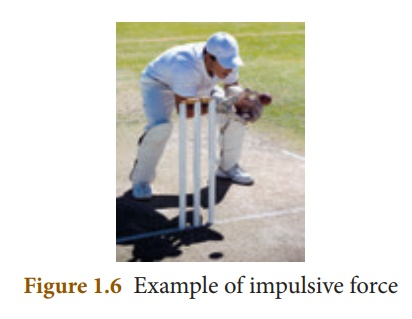Home | | Science 10th Std | Impulse

# Impulse

A large force acting for a very short interval of time is called as тАШImpulsive forceтАЩ. When a force F acts on a body for a period of time t, then the product of force and time is known as тАШimpulseтАЩ represented by тАШJтАЩ

Impulse

A large force acting for a very short interval of time is called as тАШImpulsive forceтАЩ. When a force F acts on a body for a period of time t, then the product of force and time is known as тАШimpulseтАЩ represented by тАШJтАЩ

Impulse, J = F ├Ч t (1.7)

By NewtonтАЩs second law

F = ╬Фp / t (╬Ф refers to change)

╬Фp = F ├Ч t (1.8)

From 1.7 and 1.8

J = ╬Фp

Impulse is also equal to the magnitude of change in momentum. Its unit is kg m sтАУ1 or N s. Change in momentum can be achieved in two ways. They are:

i. a large force acting for a short period of time and

ii. a smaller force acting for a longer period of time.

## Examples:

я┐╜ Automobiles are fitted with springs and shock absorbers to reduce jerks while moving on uneven roads.

я┐╜ In cricket, a fielder pulls back his hands while catching the ball. He experiences a smaller force for a longer interval of time to catch the ball, resulting in a lesser impulse on his hands.Tags : Definition, Formula, Examples , 10th Science : Chapter 1 : Laws of Motion
Study Material, Lecturing Notes, Assignment, Reference, Wiki description explanation, brief detail
10th Science : Chapter 1 : Laws of Motion : Impulse | Definition, Formula, Examples